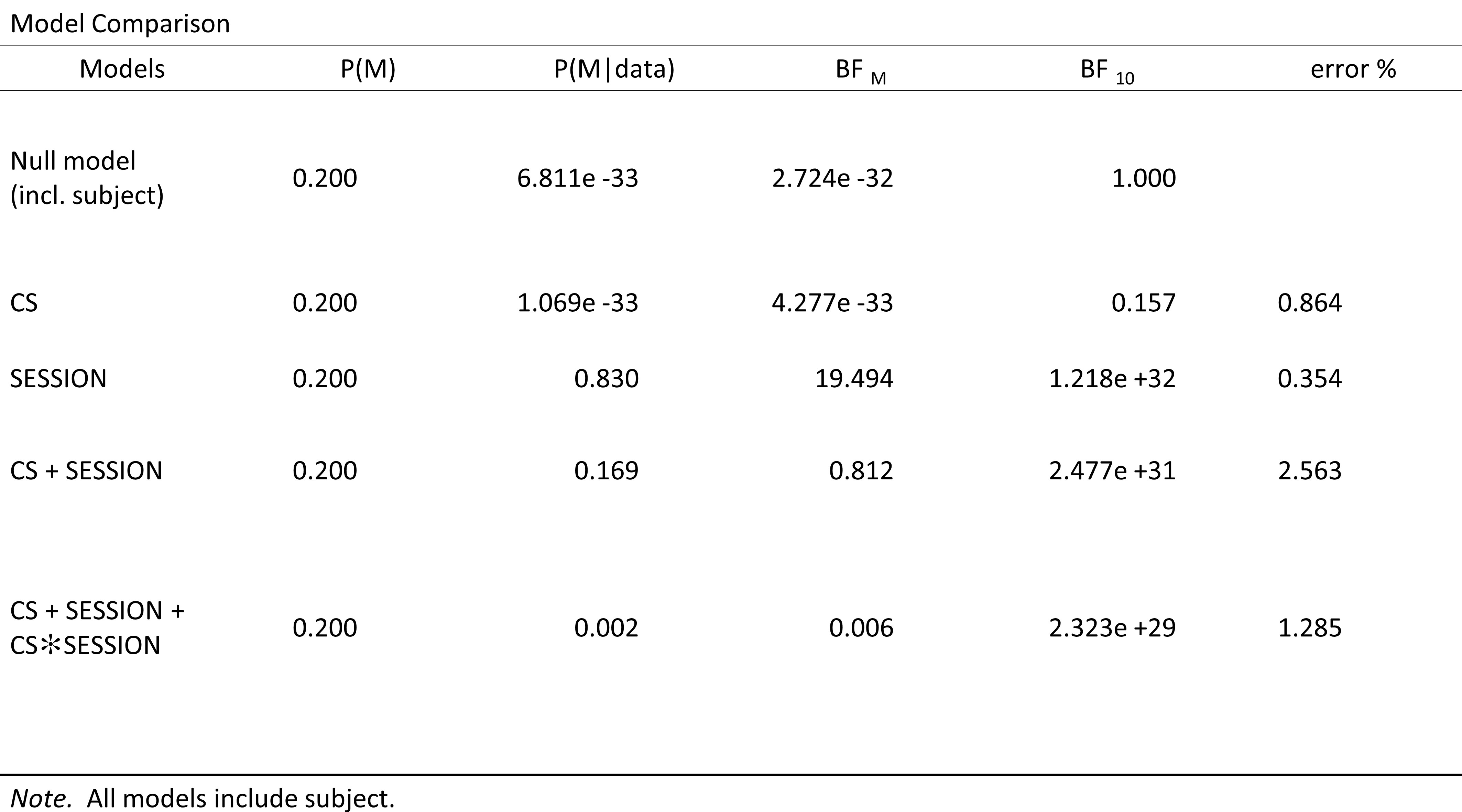Howdy, Stranger!

It looks like you're new here. If you want to get involved, click one of these buttons!

Supported by

JASP_Bayesian repeated measures ANOVA

Hi,

I am using the JASP to estimate the bayes factor. The bayesian repeated measures ANOVA was applied, however, i have a problem to get a stable bayes factor.

The results vary when i change the input names for the repeated measures factors or names of factor levels (other parameters stay the same). This issue doesnt happen when i run the repeated measure ANOVA, indicating that the data are valid.

btw, in the Advanced Options for bayesian repeated measures ANOVA, do I need to specify the samples of my experiment? or just chose the Auto option.

• Hi Rachellql,

The results in the Bayesian analysis change from one run to the next because they are obtained using a numerical approximation routine. Under Advanced Options you can increase the samples that the routine draws in order to obtain a more stable estimate. The Auto setting may sometimes give %errors that are a little to big for comfort. You should be able to up the number of samples used in the approximation routine and thereby lower the %error.

Cheers,
E.J.

• Greetings.
I have just started using JASP to run Bayesian repeated measures ANOVAs, and i have noticed some discrepancies in the values in the output table. In these cases, the BF_10 for the interaction does not correspond to what i calculate using the ratio of the p(M|data) for the interaction and the null. For example, in the table pasted below, the p for the CS*Session interaction is 0.002, and the p(H0) is 6.811E-33. By my calculation, the ratio of these is 2.936E+29, whereas the table shows the BF_10 for that model as 2.323E+29. I presume this discrepancy is a rounding error since the ratio of the p's gets closer to the BF in the table if i let p(M|data) be 0.0015 instead of 0.002.
My question then is should i use the BF values in the table in preference to the p(M|data) values if i wish to calculate the evidence for the interaction beyond the model that includes the two main effect factors? Using the probability values in the table, i get a BF (favouring no interaction) of 84.5 (=0.169/0.002), but i get a BF of 106.6 (=2.477E+31/2.323E+29) if i use the BF_10 values in the second last column.
Any advice would be greatly appreciated.
Cheers,
Justin• This must be due to rounding, in which case it is best to use the BFs. (I'll double check, thanks for bringing this up).
Cheers,
E.J.

• Great. And thanks for such a quick reply!
Justin